Courses

# Adiabatic Expansion (reversible and irreversible) Notes | EduRev

## Class 11 : Adiabatic Expansion (reversible and irreversible) Notes | EduRev

The document Adiabatic Expansion (reversible and irreversible) Notes | EduRev is a part of the Class 11 Course Chemistry for JEE.
All you need of Class 11 at this link: Class 11

In adiabatic expansion, no heat is allowed to enter or leave the system, hence, q = 0. When this value is substituted in first law of thermodynamics, ∆U= q + w, we get ∆U = w.
In expansion,

• Work is done by the system on the surroundings, hence, w is negative.
• Accordingly ∆U is also negative, i.e., internal energy decreases and therefore, the temperature of the system falls.

In case of compression,

• ∆U is positive, i.e., internal energy increases and therefore,
• The temperature of the system rises.

The molar specific heat capacity at constant volume of an ideal gas is given by.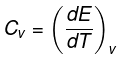dU=Cv ·dT
∆U=Cv∆T
So, w=∆U=Cv∆T
The value of ∆T depends upon the process whether it is reversible or irreversible.

Let P be the external pressure and ∆V the increase in volume. Thus, the work done by the system is
w = ∆U = – PdV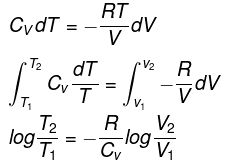We know CP – CV = R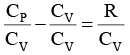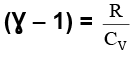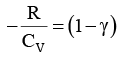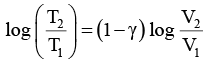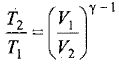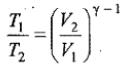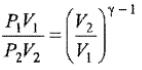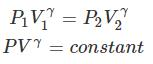Thus, knowing Ɣ, V1 , V2 and initial temperature, T1 , the final temperature, T2, can be readily evaluated.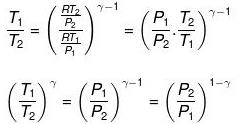Thus, knowing Ɣ, P1, P2 and initial temperature, T1, the final temperature, T2, can be readily evaluated.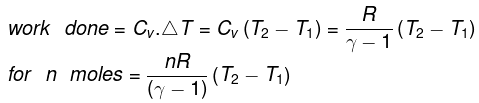In free expansion, the external pressure is zero, i.e. , work done is zero. Accordingly, ∆U which is equal to w is also zero. If ∆U  is zero, ∆T should be zero. Thus, in free expansion (adiabatically),
∆T = 0, ∆U= 0, w = 0 and ∆H = O.
In intermediate expansion, the volume changes from V1 to V2 against external pressure, Pext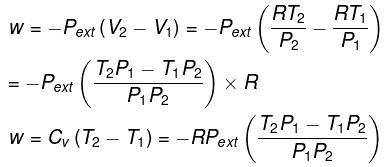or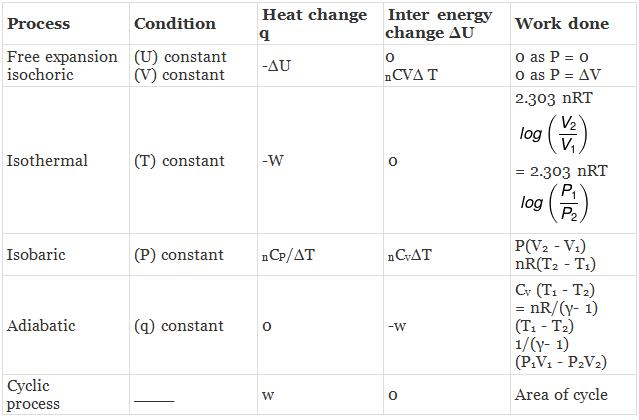Example 1. Two moles of an ideal monoatomic gas at NTP are compressed adiabatically and reversibly to occupy a volume of 4.48 dm3. Calculate the amount of work done, ∆U, final temperature and pressure of the gas. Cv for ideal gas 12.45J K -1 mol-1.
Solution. For an ideal gas,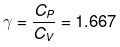Initial volume, V= 2 × 22.4 = 44.8 dm3
Initial pressure, P1 = 1 atm
Initial temperature, T1 = 273 K
Final volume, V2 = 4.48 dm3
Let the final pressure be Pand temperature be T
Applying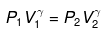or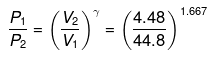or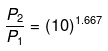P2 = (10)1.667(P1 = 1 given)
log P2 = 1.667 log 10= 1.667
P2 = antilog 1.667= 46.45 atm
Final temperature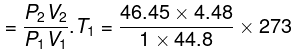= 1268 K

Work done on the system = n.Cv.ΔT
= 2 × 12.45 × (1268 - 273)
= 2 × 12.45 × 995 = 24775.5 J
From the first law of thermodynamics,
ΔE = q + w = 0 + 24775.5 = 24775.5 J

Example 2. A certain volume of dry air at NTP is expanded reversibiy to four times its volume (a) isothermally (b) adiabatically. Calculate thefinal pressure and temperature in each case, assuming ideal behaviour.
(CP \ CV for air = 1.4)
Solution.
Let V1 be the initial volume of dry air at NTP.
(a) Isothermal expansion: During isothermal expansion, the temperature remains the same throughout. Hence, final temperature will be 273 K.
Since P1V1 = P2V2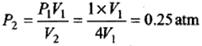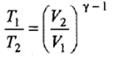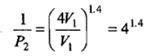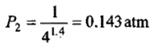CALCULATION OF ∆H, ∆U. WORK, HEAT ETC.
Case - 1 For an ideal gas undergoing a process. the formula to be used are: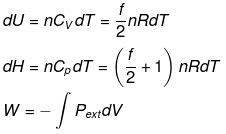dH = dU + d(PV)
ΔH = ΔU + nRΔT
du = dQ + dw

Calculation of q, w , ∆H, ∆U for a reversible isothermal process involving an ideal gas :
ΔU = q + w = 0 ⇒ -w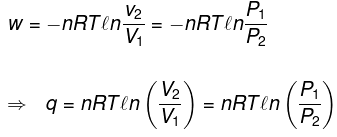Calculation of q, w, ∆H, ∆U for an Irreversible isothermal process involving an ideal gas:
For isothermal process involving
ΔH = ΔU = 0 ∵ ΔT = 0
Also,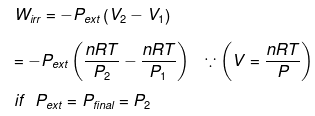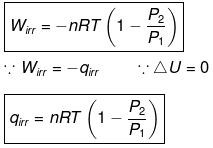For isobaric process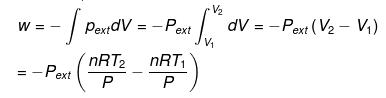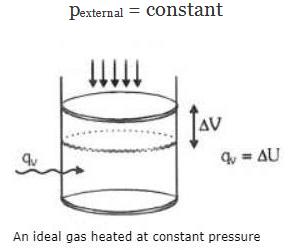= -nR(T2 - T1) (∵ Pext = P)
= -nRΔT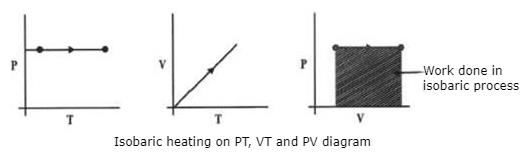Calculation of q, w, ∆H, ∆U for an IRREVERSIBLE ISOCHORIC process involving an ideal gas: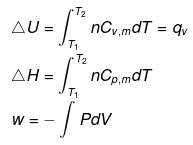w= 0   ∵ dV = 0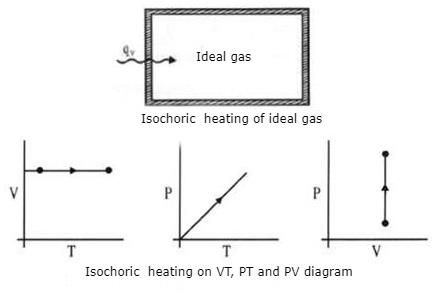Calculation of q, w, ∆H, ∆U for reversible adiabatic process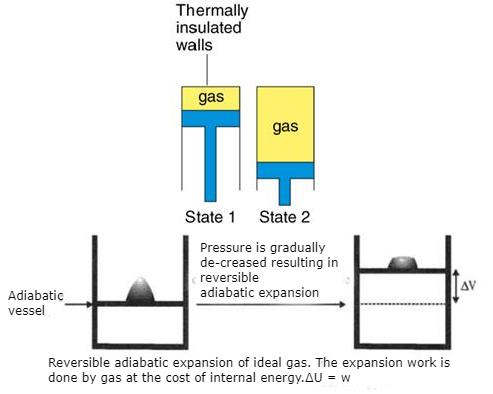For an adiabatic process,
dq = 0 ⇒ dU = dw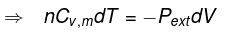for a reversible change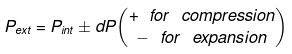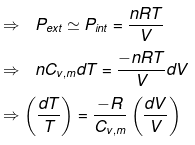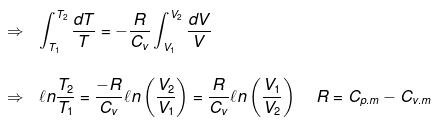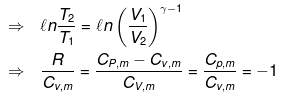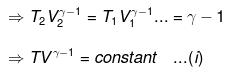Now substituting V = nRT/P in equation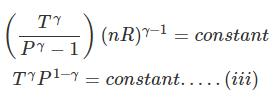substituting T = PV/nR in eq...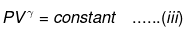⇒ Equation (i), (ii) and (iii) is valid only for reversible adiabatic process, for irreversible adiabatic process these equations are not applicable.

• Expression for work: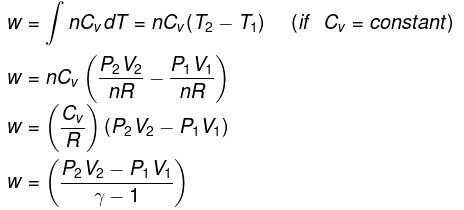• Expression for ΔH and ΔU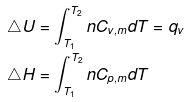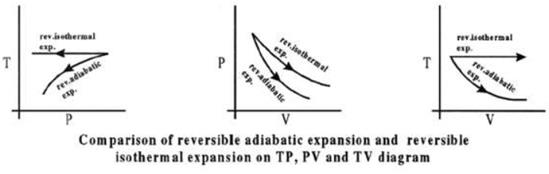CALCULATION OF Q, W, ∆H, ∆U FOR IRREVERSIBLE ADIABATIC
PROCESS INVOLVING AN IDEAL GAS:
Operation wise adiabatic process and isothermal process are similar hence all the criteria that is used for judging an isothermal irreversible process are applicable to adiabatic process.

If large amount of dust particles are removed abruptly an irreversible adiabatic expansion take place.

In an irreversible adiabatic process, an ideal gas is subjected to compression or expansion in a thermally insulated vessel.

The heat absorbed in the process =0
⇒ dU = wirr ....(i)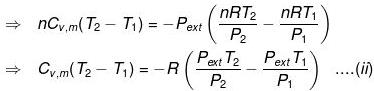If Pext, = P2 = Pfinal
Then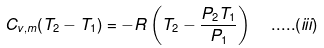eq. (ii) or (iii) can be solved for T2
Expression for wNote: If two states A and B are connected by a reversible path then they can never be connected by an irreversible path.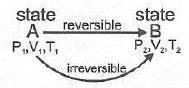If the two states are linked by an adiabatic reversible and irreversible path then wrev = ∆Urev
But as U is a state function
Therefore, ∆U irrev = ∆Urev
wirrev = wrev
as work is a path function.

If we assume that
wirrev = wrev

It implies that   which again is a contradiction as U is a state function.
∆U irrev ≠ ∆Urev
Two states A and B can never lie both on a reversible as well as irreversible adiabatic path.

There lies only one unique adiabatic path linkage between two states A and B.

Offer running on EduRev: Apply code STAYHOME200 to get INR 200 off on our premium plan EduRev Infinity!

## Chemistry for JEE

223 videos|446 docs|334 tests

,

,

,

,

,

,

,

,

,

,

,

,

,

,

,

,

,

,

,

,

,

;